Next: Constraints for the scalar-isoscalar Up: Continuity equations in the Previous: Continuity equations in the

## Constraints for zero-order terms

For zero-order terms, condition (48) gives no constraints on the coupling constants, apart from those in the vector-isovector channel, where we have,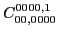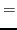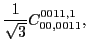(51)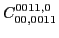(52)

whereas the coupling constant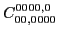is unrestricted.

It is interesting to discuss these results in connection with the derivation of the CE for a spin-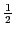particle moving in a local potential, which we gave in Sec. 2.1. There, we pointed out that the CE in the vector channel is valid when the vector potential is parallel to the spin density. Exactly this situation occurs for the zero-order EDF, where in each spin-isospin channel the potential functions are simply proportional to densities.

In fact, the simple algebraic rule of the vector product in Eq. (5) is equivalent to the coupling of pairs of identical commuting rank 1 tensors to rank 1, which is identically null. This is just the case for the coupling to rank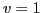(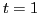) in the spin (isospin) space for the vector-isoscalar (scalar-isovector) channel of the CE, in which the identically null tensors formed by the pairs of densities at zero order leave the corresponding coupling constants unrestricted. However, when the coupling to a rank-1 tensor is simultaneously performed in both spin and isospin space (and), as is the case in the vector-isovector channel of the CE, the two negative signs in the commutation of the pair of densities give an overall positive sign, and the above selection rule does not apply. This explains why, at zero order of the vector-isovector channel, condition (48) does induce constraints on coupling constants - those given in Eqs. (51) and (52).Next: Constraints for the scalar-isoscalar Up: Continuity equations in the Previous: Continuity equations in the
Jacek Dobaczewski 2011-11-11